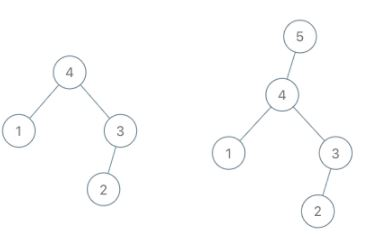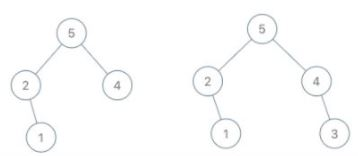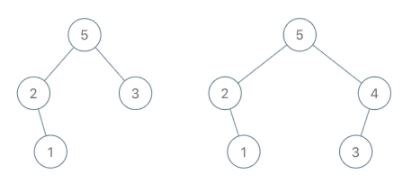# 998. Maximum Binary Tree II

Medium
A maximum tree is a tree where every node has a value greater than any other value in its subtree.
You are given the `root` of a maximum binary tree and an integer `val`.
Just as in the previous problem, the given tree was constructed from a list `a` (`root = Construct(a)`) recursively with the following `Construct(a)` routine:
• If `a` is empty, return `null`.
• Otherwise, let `a[i]` be the largest element of `a`. Create a `root` node with the value `a[i]`.
• The left child of `root` will be `Construct([a, a, ..., a[i - 1]])`.
• The right child of `root` will be `Construct([a[i + 1], a[i + 2], ..., a[a.length - 1]])`.
• Return `root`.
Note that we were not given `a` directly, only a root node `root = Construct(a)`.
Suppose `b` is a copy of `a` with the value `val` appended to it. It is guaranteed that `b` has unique values.
Return `Construct(b)`.
Example 1:Input: root = [4,1,3,null,null,2], val = 5
Output: [5,4,null,1,3,null,null,2]
Explanation: a = [1,4,2,3], b = [1,4,2,3,5]
Example 2:Input: root = [5,2,4,null,1], val = 3
Output: [5,2,4,null,1,null,3]
Explanation: a = [2,1,5,4], b = [2,1,5,4,3]
Example 3:Input: root = [5,2,3,null,1], val = 4
Output: [5,2,4,null,1,3]
Explanation: a = [2,1,5,3], b = [2,1,5,3,4]
Constraints:
• The number of nodes in the tree is in the range `[1, 100]`.
• `1 <= Node.val <= 100`
• All the values of the tree are unique.
• `1 <= val <= 100`

### 解題

/**
* Definition for a binary tree node.
* type TreeNode struct {
* Val int
* Left *TreeNode
* Right *TreeNode
* }
*/
func insertIntoMaxTree(root *TreeNode, val int) *TreeNode {
if root == nil {
return &TreeNode{ Val:val }
}
if val > root.Val {
newnode := &TreeNode { Val:val, Left: root}
return newnode
}
root.Right = insertIntoMaxTree(root.Right, val)
return root
}